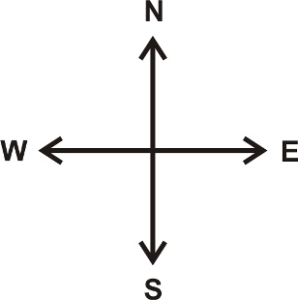# Introduction to Direction | The Significance of Four Cardinal Points

### LESSON – INTRODUCTION TO DIRECTION

TOPIC

1. Introduction

2. Meaning of Cardinal Points

3. The Importance of Four Cardinal Points

INTRODUCTION

Direction is the position along which someone or something moves or faces.

Direction is used to describe or determine the position of someone or something. Sometimes, it is used as right , left, forward (up) or backward (down).

Geographically, north, south, east and west are used in describing directions.

This is called cardinal points.

FOUR CARDINAL POINTSNorth, east, south, and west are the four cardinal directions that’s marked by the initials N, E, S, and W.

1. North

2. South

3. East

4. West

IMPORTANCE OF FOUR CARDINAL POINTS

The significance of four cardinal points are as follows:

1. It is used to calculate time.

2. It is used to locating places.

3. It is used for directions.

4. It is used for tracking someone or something.

LESSON EVALUATION

1. describe the four cardinal points.

2. mention 4 points on the cardinal points.

3. state 4 significance of four cardinal points.

4. draw and indicate the four cardinal points.

1.•